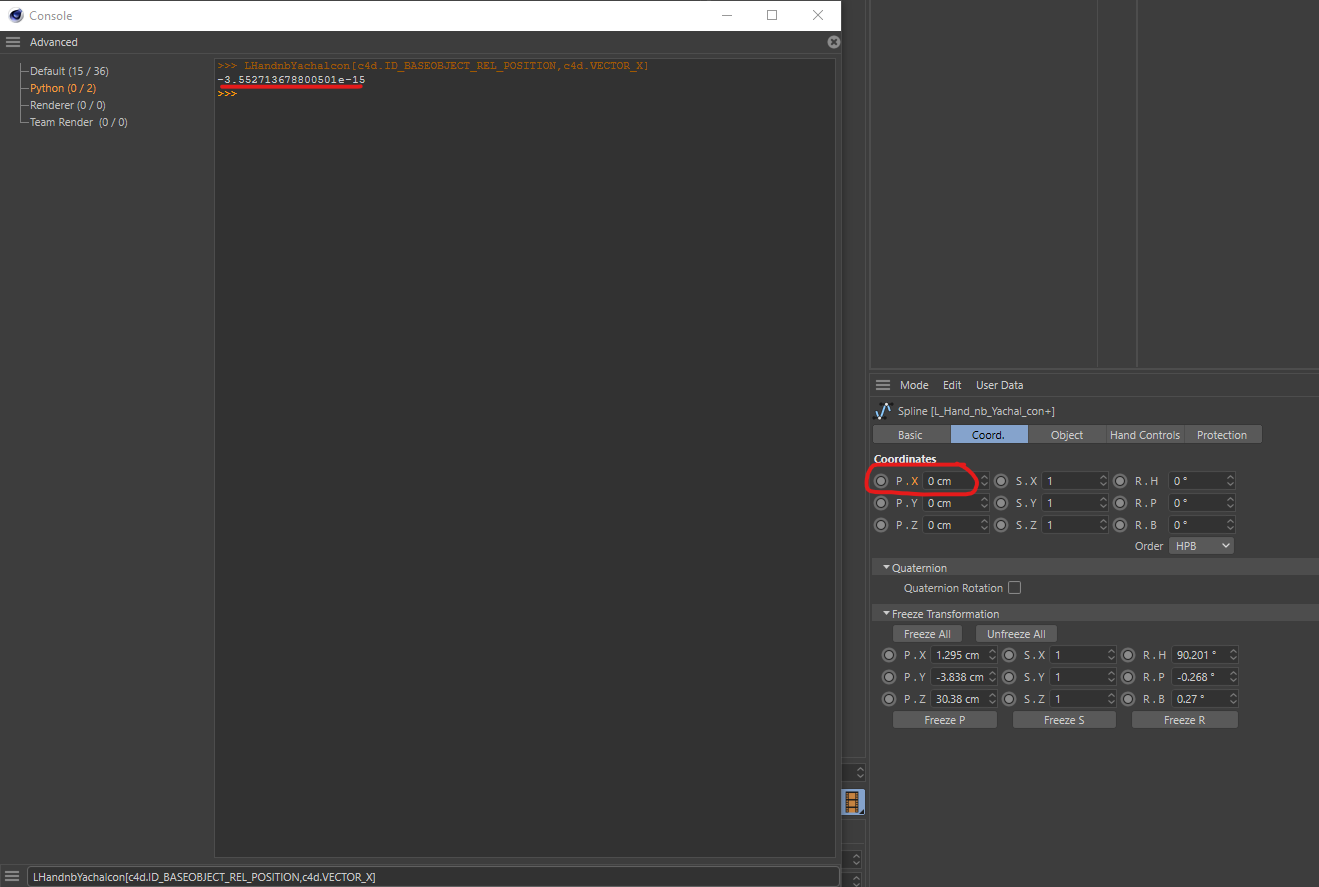# SOLVEDWhy are my Relative Transformation Values not 0?

Hello,
I'm having an issue where I'm trying to compare an object's relative transformation Vectors to another Vector.

As an example, if I print the values as such, I get a Vector with 0 values, just as can be seen in the Attributes Editor:

``````print obj[c4d.ID_BASEOBJECT_REL_POSITION]
#Vector(0, 0, 0)
``````

If I compare the relative Position to a Vector with 0 values, however, it returns as `False`.

``````print obj[c4d.ID_BASEOBJECT_REL_POSITION] == c4d.Vector(0, 0, 0)
#False
``````

If I print the Position.x it returns an unexpected number:

``````print obj[c4d.ID_BASEOBJECT_REL_POSITION,c4d.VECTOR_X]
#-3.552713678800501e-15
``````

Can anyone explain what is happening? My objects do have frozen transformation values, but it seems like the relative values should ignore these.Thank you.

Hi,

there is `c4d.utils.CompareFloatTolerant(a, b)` although the function is a bit of a black-box. You could also write your function, the problem here is that you might run into floating point precision problems depending on how accurate you want that function to be. The utils function is addressing that problem.

``````COMPARE_DELTA = 1e-10

a = 0
b = 3.1415e-14

print "a:", a, "b:", b
print "a == b:", a == b

tolerant_compare = lambda a, b: abs(a - b) <= COMPARE_DELTA

print "tolerant compare:", tolerant_compare(a, b)
``````
``````a: 0 b: 3.1415e-14
a == b: False
tolerant compare: True
``````

edit: yes, the stack overflow snippet addresses your problem, it is a slightly fancier version of my lambda.

FYI: If you are not interested only in a single component of a vector, you can also always evaluate the squared length of the difference vector of the two vectors you want to compare.

Cheers,
zipit

#-3.552713678800501e-15

That is zero, at least as far as computer scientists programmers are concerned. That is a number with 14 zeros after the comma and then 3552 etc. The reason why this is happening is floating point precision.

Cheers,
zipit

@zipit Thank you. So how do I compare it to 0? Currently it does not equate to zero.

I found this solution on StackOverflow

``````def isclose(a, b, rel_tol=1e-09, abs_tol=0.0):
return abs(a-b) <= max(rel_tol * max(abs(a), abs(b)), abs_tol)
``````

This seems to address the issue.

I am still confused as to why the Vectors do not compare equally though:

``````print obj[c4d.ID_BASEOBJECT_REL_POSITION] == c4d.Vector(0, 0, 0)
#False
``````

It seems like the floating point issues would be addressed in `Vector.__eq__` and `Vector.__ne__`

Hi,

there is `c4d.utils.CompareFloatTolerant(a, b)` although the function is a bit of a black-box. You could also write your function, the problem here is that you might run into floating point precision problems depending on how accurate you want that function to be. The utils function is addressing that problem.

``````COMPARE_DELTA = 1e-10

a = 0
b = 3.1415e-14

print "a:", a, "b:", b
print "a == b:", a == b

tolerant_compare = lambda a, b: abs(a - b) <= COMPARE_DELTA

print "tolerant compare:", tolerant_compare(a, b)
``````
``````a: 0 b: 3.1415e-14
a == b: False
tolerant compare: True
``````

edit: yes, the stack overflow snippet addresses your problem, it is a slightly fancier version of my lambda.

FYI: If you are not interested only in a single component of a vector, you can also always evaluate the squared length of the difference vector of the two vectors you want to compare.

Cheers,
zipit

@zipit Your lambda is working better for my current project than the StackOverflow function. I assume this is because of the tolerance? Regardless, thank you very much for your help!!

Jeah, mine is rather tolerant, it bascially ignores everything after the 9th digit. So use with care@zipit Will do. Thank you!

Hi @blastframe just to clarify how c4d.utils.CompareFloatTolerant works, this actually performs a check based on the bit representation.
It's not 100% safe but would work in most of Cinema 4D case and it's way faster than a real comparison.

Cheers,
Maxime.

Hi,

what I meant with "is a bit of a black box" was, that it remains unclear to me, what the function considers "sufficiently close to each other". Does that mean it mimics the rounding behavior of the Cinema 4D app or does it mean that it analyzes the bit-patterns of the operands for avalanche patterns that indicate a precision error?

I did not want to imply that it is broken, because I never actually bothered using or testing it, because of the uncertainty of its description.

Cheers,
zipit

The source code of the C++ version of `CompareFloatTolerant()` is actually available in the `frameworks\cinema.framework\source\ge_ieee.cpp` and/or `\frameworks\core.framework\source\maxon\general_math.cpp` file of the C++ SDK. I assume the Python version just calls the C++ function.